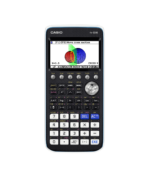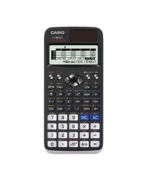Equations Archives - Casio Calculators

# Equations

Displaying Resources

FilterHow to Video
fx-CG50 A-Level GCSE IB
Quick Skill: Solving Linear Equations

How to solve a linear equation using the numerical SolveN function....How to Video
fx-CG50 A-Level GCSE IB
Quick Skill: Simultaneous Equations – Using Equation Solutions

How to extract the solutions to a system of linear simultaneous equations found ...How to Video
fx-CG50 A-Level GCSE IB
Quick Skill: Polynomials – Using Equation Results

How to extract a polynomial's solutions found using the Equation app. You will n...How to Video
fx-CG50 A-Level GCSE IB
Simultaneous Equations – Solving Numerically

How to use Equation to solve simultaneous linear equations in two variables nume...How to Video
fx-CG50 A-Level
Simultaneous Equations – Solving Harder Equations Graphically

How to solve a simultaneous linear and quadratic equation in both x and y graphi...How to Video
fx-CG50 A-Level GCSE IB
Simultaneous Equations – Solving Graphically

How to solve two simultaneous linear, or linear and quadratic, equations using g...How to Video
fx-CG50 A-Level GCSE IB

How to use Equation to numerically solve a polynomial equation with real roots....How to Video
fx-CG50 A-Level IB
Graphing – Types of Function

Plot graphs of parametric functions and where x = constant. Plot inequalities an...How to Video
A-Level GCSE
Solving Polynomials

How to solve polynomials of order 2, 3 or 4 with exact values. Find vertex of a ...How to Video
GCSE A-Level
Solve Function

How to solve equations numerically by inputting a start value for each solution....How to Video
A-Level GCSE
Simultaneous Equations

How to solve linear simultaneous equations. Rearrange equations to correctly inp...How to Leaflet
fx-CG50 A-Level GCSE IB
Simultaneous Equations – Solving Numerically (Worksheet)

Worksheet to accompany the video on how to use Equation to solve simultaneous li...How to Leaflet
fx-CG50 A-Level GCSE IB
Quadratic Equations – Solving Numerically (Worksheet)

Worksheet to accompany the video on how to use Equation to numerically solve a p...How to Leaflet
fx-CG50 A-Level
Graphing – Types of Function (Worksheet)

Worksheet to accompany the video on how to plot graphs of parametric functions a...How to Leaflet
fx-CG50 A-Level GCSE IB
Equation Quick Start fx-CG50

How to solve polynomial equations of order 2 and higher on the fx-CG50....How to Leaflet
fx-991EX A-Level GCSE
Equation Quick Start fx-991EX

How to solve simultaneous equations with up to 4 unknowns. Solve polynomial equa...How to Leaflet
A-Level GCSE IB
Equation Quick Start fx-9860GII

How to solve polynomial equations of order 2 and higher on the fx-9860GII. Solve...How to Leaflet
fx-CG50 A-Level
Sequences – Recursion and Equations (Worksheet)

Worksheet to accompany the video on how to iterate using ANS to generate terms i...How to Leaflet
fx-991EX A-Level GCSE
Inequality Quick Start fx-991EX

How to solve quadratic, cubic and quartic inequalities....How to Video
fx-CG50 A-Level
Sequences – Recursion and Equations

How to iterate using ANS to generate terms in arithmetic and geometric sequences...